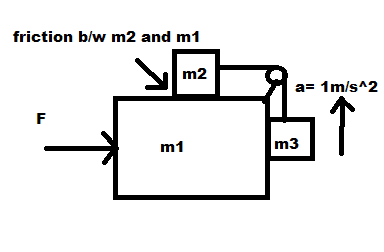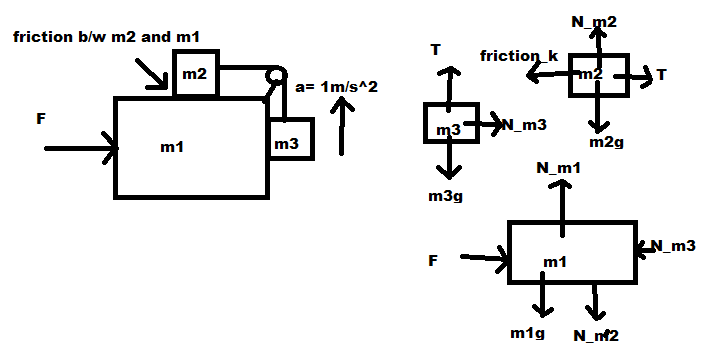# FBD forces check my work please

shizaep

## Homework Statement

1)What should F be so that m3accelerates upwards at 1 m/s2?F = ma

## The Attempt at a Solution

So I start by drawing FBD's for everything:^^also, there is supposed to be a force of friction to the right on M1, I factored that into my solution but forgot to draw in in the diagram.

Then I proceed to sum the x and y components of the forces where it is relevant.
m3:

ƩFy=m3a1
T-m3g=m3a1

m2:
μkm2g-T=m2a1

m1:

F-Nm3km2g=m1a2
F=(m1+m3)(a2-1)-μkm2g

i got the relationship a2=a1-1
(a2is the acceleration of the big block and a1 is the acceleration of the 2 smaller blocks)

For a final answer I got:

F = (m1+m3)[(μkm2g-m3g)/(m2+m3)-1]-μkm2g

I am not sure if this is correct. May someone verify this solution please. In particular, I was unsure about the contact forces between the large block and the block accelerating vertically.

Last edited:

## Answers and Replies

NihalSh
you haven't mentioned if friction exists and if yes at which all contact surfaces?.........

according to your diagram, friction acts on m2, then due to Newton's Third Law equal in magnitude but opposite in direction friction force must act on m1 (which you missed).

Edit: I guess, I didn't look carefully enough. Your diagram mentions that friction force exists between m1 and m2. What about other surfaces?......I assume you have drawn the diagram from the textbook/assignment, so friction doesn't exist anywhere else.

Last edited:
shizaep
oh ya i forgot that complimentary frictions must act on M1. brb will edit that into my solution.

also, for this example, you can assume that there is only friction between M1 and M2, nowhere else.

NihalSh
oh ya i forgot that complimentary frictions must act on M1. brb will edit that into my solution.

also, for this example, you can assume that there is only friction between M1 and M2, nowhere else.

okay. balance the forces for all the three masses, you'll arrive at the answer. If you face problem, post again!!

shizaep
I added the force of friction on M1. (to the right)
My final answer makes sense to me in my mind but I am not sure if there is some kind of mistake or something that I am missing.
(am I correct to say that Nm3which acts on block M1 is m3a2?)

NihalSh
I added the force of friction on M1. (to the right)(am I correct to say that Nm3which acts on block M1 is m3a2?)

Check your FBD again, I notice that their is a problem with direction of friction mentioned for block m2. Since m3 is accelerating upwards that means m2 should accelerate towards the left wrt to block m1. That means friction should act towards right on block m2 or left on m1.

(am I correct to say that Nm3which acts on block M1 is m3a2?)

yes, you are correct about that because normal force on m3 accelerates it towards right along with m1, so both have same horizontal acceleration

if you do the corrections to your FBD, you'll notice that block m2 has all the horizontal forces toward right but it accelerates towards left with respect to m1. you'll have to find relation between relative acceleration of "m2 with respect to the block m1" and "m2 with respect to ground"

I mention to make sure that it is clear, every block has a different net (vector) acceleration but it is all correlated.

P.S. :

##a_{m2}## is acceleration of m2 with respect to ground
##a_{1}## is acceleration of m1 with respect to ground
##a_{2}=1 m/s^2## is acceleration of m2 with respect to the block m1

##a_{m2}= a_{1}-a_{2}## .........minus sign means towards the left

$$a_{m1}= a_{1}$$
$$a_{m3x}= a_{1}$$
$$a_{m3y}= a_{2}$$

Last edited: